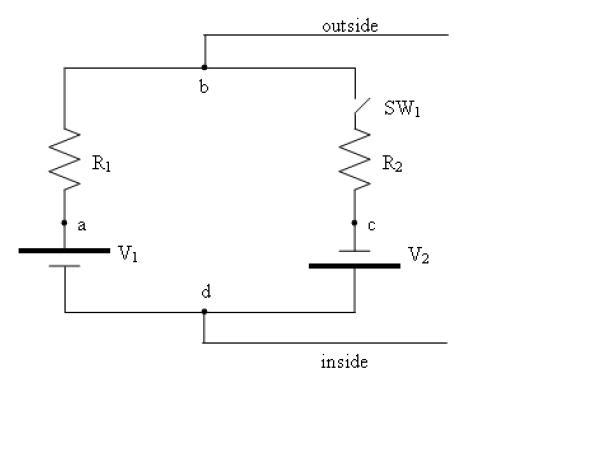# Circuits question

muscles00gt
Sorry if this is in the wrong area, but I'm having a bit of difficulty on one of my homework problems.

A simple model of the membrane of a nerve cell is shown in the figure at the right (seen below). It consists of 2 batteries (ion pumps) with voltages V1=100mV and V2=50mV. The resistance to flow across the membrane is represented by two resistors with resistances R1=10KΩ and R2=90KΩ. The variability is represented by a switch, SW1. Four points are labeled by the letters a-d. Point b represents outside of membrane and point d inside the membrane.

Picture of circuit:A) What is the voltage difference across the membrane when the switch is open?

B) What is the current flowing around the loop when the switch is closed?

C) What is the voltage drop across the resistor R1 when switch is open? closed?

D) What is the voltage drop across the resistor R2 when switch is open? closed?

E) What is the voltage difference across the membrane when switch is closed?

F) If the locations of resistance R1 and R2 were reversed, would the voltages across the cell membranes be different?